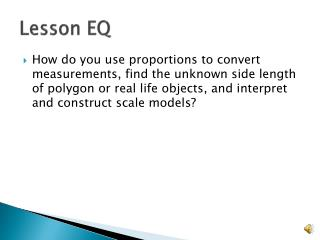DownloadDownload PresentationLesson EQ

# Lesson EQ

Télécharger la présentation## Lesson EQ

- - - - - - - - - - - - - - - - - - - - - - - - - - - E N D - - - - - - - - - - - - - - - - - - - - - - - - - - -
##### Presentation Transcript

1. Lesson EQ • How do you use proportions to convert measurements, find the unknown side length of polygon or real life objects, and interpret and construct scale models?

2. Converting Units • We can also use cross products to solve proportions to convert units. • In order to do this we must know the units, though. • Please copy the following two slides neatly into your work. • You will be quizzed separately on each set of information.

3. Customary System Measurements • Length: • 12 inches = 1 foot • 3 feet = 1 yard • 5280 yards = 1 mile • Weight: • 16 oz = 1 lb • 2000 lb = 1 ton • Capacity • 8 fl oz = 1 cup • 2 cups = 1 pint • 2 pints = 1 quart • 4 quarts = 1 gallon

4. Metric System • 1 millimeter = 0.001 meter • 1 centimeter = 0.01 meter • 1 decimeter = 0.1 meter • 1 meter • 1 dekameter = 10 meters • 1 hectometer = 100 meters • 1 kilometer = 1,000 meters

5. Analyze Units • To analyze units we will be given a value in one unit and be told to change it into a different unit. • We will set these units as a ratio. • We will set that ratio equal to a ratio from our measurement notes. • We will solve for the missing value. • Make sure that the units match in each ratio.

6. Analyze Units • The average American eats 23 pounds of pizza per year. Find the number of ounces of pizza the average American eats per year. • 23 lbx oz • We know 16 oz = 1 lb. Set up a proportion • 23 lb = 1 lb x oz 16 oz • Cross multiply: 23 • 16 = 1x • Simplify: 368 oz

7. Analyze Units • Aihua drinks 4 cups of water a day. Find the total number of fluid ounces of water she drinks per day. • 4 cupsx fl oz • 8 fl oz = 1 cup ~ Set up a proportion • 4 cups = 1 cupx fl oz 8 fl oz • Cross multiply: 4 • 8 = 1x • Simplify: 32 fl oz

8. Analyze Units • A model airplane flies 22 feet in 2 seconds. How many inches did the airplane fly? • 22 ftx in • 12 inches = 1 foot ~ set up proportion • 22 ft = 1 ftx in 12 in • Cross multiply: 22 • 12 = 1x • Simplify: 264 inches

9. Your Turn • A fish swims 13 centimeters. How many meters did the finish swim? • 13 cm x m • 1 cm = 0.01 m ~ set up a proportion • 13 cm = 1 cmx m 0.01m • Cross multiply: 13 • 0.01 = 1x • Simplify = 0.13 m

10. Try Another • 8 gallons equals how many quarts? • 8 galx qt • 4 quarts = 1 gallon • 8 gal = 1 galx qt 4 qt • Cross multiply: 8 • 4 = 1x • Simplify 32 quarts